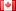# Revision history [back]

### Factoring bivariate polynomials w.r.t. a single variable

the function factor() works in this fashion:

sage: x, y = PolynomialRing(GF(17), 2, ['x','y']).gens()

sage: f = 9y^6 - 9x^2y^5 - 18x^3y^4 - 9x^5y^4 + 9x^6y^2 + 9x^7y^3\ ... + 18x^8y^2 - 9x^11

sage: f.factor()

(-9) * (x^5 - y^2) * (x^6 - 2x^3y^2 - x^2*y^3 + y^4)

Is there a possibility to factorize a bivariate polynomial in x,y with respect to a single variable only (e.g. y) and get the answer in the form of (y - f(x)) as factors?2 No.2 RevisionDSM5242 ●21 ●68 ●112

### Factoring bivariate polynomials w.r.t. a single variable

the function factor() works in this fashion:

sage: x, y = PolynomialRing(GF(17), 2, ['x','y']).gens()['x','y']).gens()
sage: f =  9y^6 9*y^6 - 9x^2y^5 9*x^2*y^5 - 18x^3y^4 18*x^3*y^4 - 9x^5y^4 9*x^5*y^4 + 9x^6y^2 9*x^6*y^2 + 9x^7y^3\
...   9*x^7*y^3 + 18x^8y^2 18*x^8*y^2 - 9x^119*x^11
sage: f.factor()
sage: f.factor() (-9) * (x^5 - y^2) * (x^6 - 2x^3y^2 2*x^3*y^2 - x^2*y^3 + y^4)y^4)


Is there a possibility to factorize a bivariate polynomial in x,y with respect to a single variable only (e.g. y) and get the answer in the form of (y - f(x)) as factors?3 retaggedFrédéricC4385 ●3 ●37 ●93

### Factoring bivariate polynomials w.r.t. a single variable

the function factor() works in this fashion:

sage: x, y = PolynomialRing(GF(17), 2, ['x','y']).gens()

sage: f =  9*y^6 - 9*x^2*y^5 - 18*x^3*y^4 - 9*x^5*y^4 + 9*x^6*y^2 + 9*x^7*y^3 + 18*x^8*y^2 - 9*x^11

sage: f.factor()

(-9) * (x^5 - y^2) * (x^6 - 2*x^3*y^2 - x^2*y^3 + y^4)


Is there a possibility to factorize a bivariate polynomial in x,y with respect to a single variable only (e.g. y) and get the answer in the form of (y - f(x)) as factors?